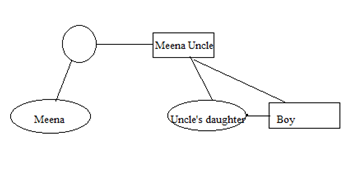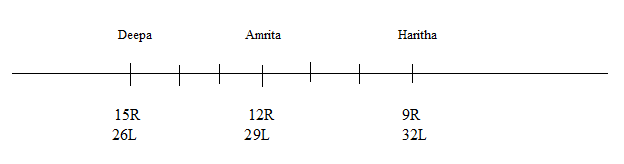# SSC CGL 2018 Practice Test Papers | Reasoning Ability (Day-44)

Dear Aspirants, Here we have given the Important SSC CGL Exam 2018 Practice Test Papers. Candidates those who are preparing for SSC CGL 2018 can practice these questions to get more confidence to Crack SSC CGL 2018 Examination.

[WpProQuiz 3353]

Click “Start Quiz” to attend these Questions and view Explanation

1) Select the related world/letters/numbers/ figures from the given alternatives.

100: 20:144:?

a) 25

b) 30

c) 22

d) 24

2) A series is given, with one term missing. Choose the correct alternative from the given ones that will complete the series.

25, 7, 27, 9, 33, 6, 42, ?

a) 5

b) 6

c) 7

d) 8

3) Pointing towards a boy Meena said, “He is the brother of the only daughter of my maternal uncle.” How is that boy related to Meena?

a) Uncle

b) Brother

c) Cousin

d) Nephew

4) From the given alternatives select the word which cannot be formed using the letters of the given word

b) NET

c) LID

d) RAM

5) Select the correct combination of mathematical signs to replace * signs and to balance the following equation:

8 * 3 * 12 * 12

a) ÷ – =

b) + – ÷

c) = – ÷

d) × – =

6) In a row of 40 children, Haritha is sixth to the right of Deepa who is fifteenth from the right end. What is the position of Amritha from Deepa who is third to left of Haritha?

a) Third to the right

b) Third to the left

c) Second to the right

d) Second to the left

7) In a row of 40 children, Haritha is sixth to the right of Deepa who is fifteenth from the right end. What is the position of Amritha from Deepa who is third to left of Haritha?

a) 30th

b) 28th

c) 29th

d) 31st

Six persons A, B, C, D, E and F are sitting in a straight line facing north. D and A are sitting end of the row. B is to the immediate left of E and C is to the right of E and F is to the left of A and is not a neighbor of C.

8) What is the position of B with respect to C?

a) Second to the right

b) Second to the left

c) Third to the right

d) Third to the left

9) Who among the following sits between D and B?

a) F

b) F, C

c) C, E, B

d) A

10) If B is replaced by a new person M what is the position of M from right end?

a) fourth

b) Third

c) Second

d) First

√100+10=20

√144+10=22

(25, 7) = 2+5 = 7

(27, 9) = 2+7 = 9

(33, 6) = 3+3 = 6

(42, ?) = 4+2 = 6M is not there in the given word.

8×3 – 12 = 12Direction (8-10) :

Arrangement is DFBECA

Arrangement is DFBECA